Browse by Topic

# New Methods for Computing Algebraic Integrals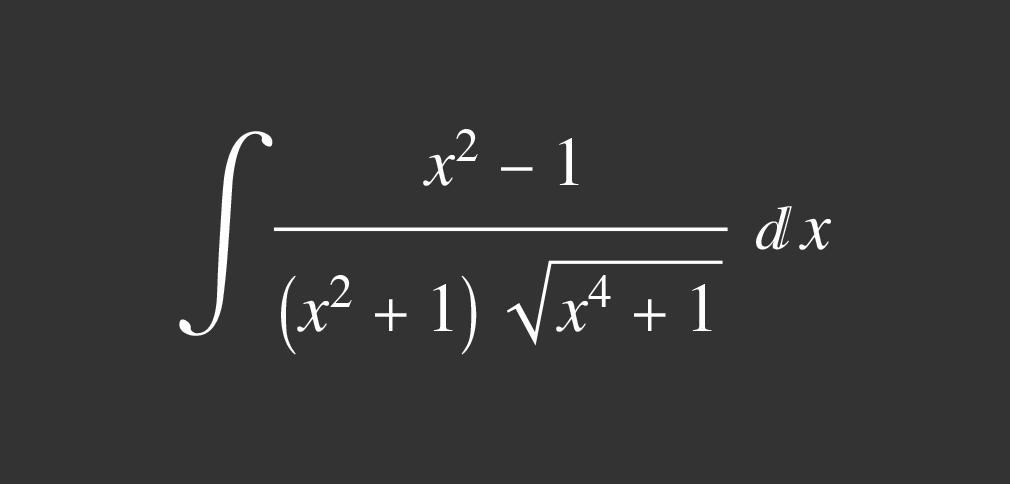In 2004, I became obsessed with computing integrals, using both elementary techniques known to calculus students and the Risch algorithm and its extensions by Davenport, Trager and Bronstein [1, 2, 3, 4, 5]. Taking inspiration from George Beck’s step-by-step differentiation program, WalkD, I decided to write a step-by-step program for computing indefinite integrals.

For example, it’s easy to write a simple set of replacement rules in Mathematica for integrating (expanded) polynomials: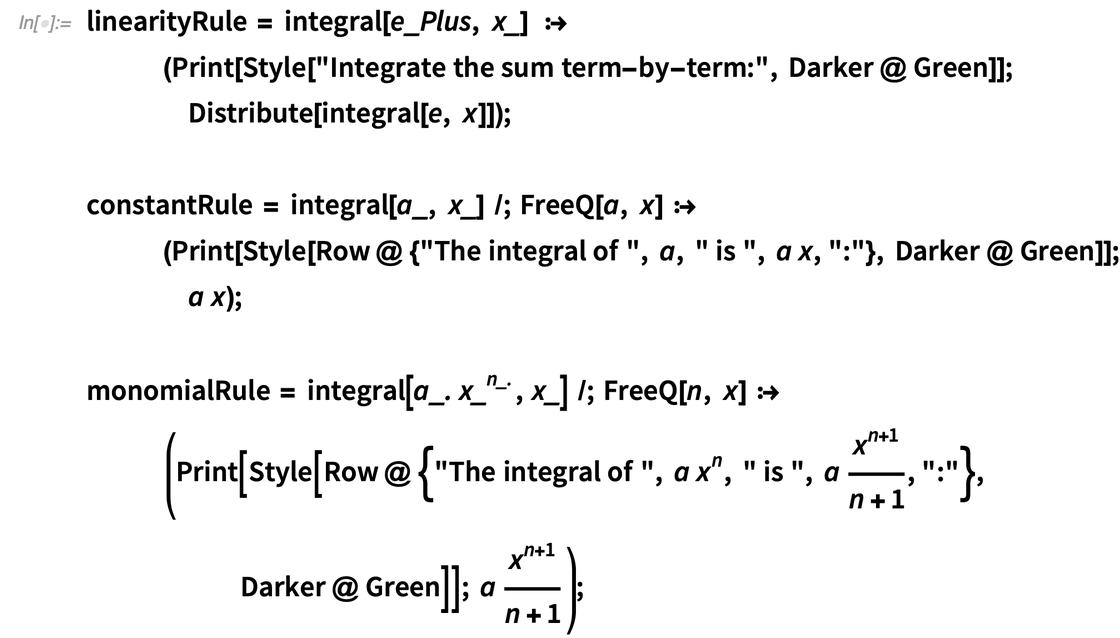✕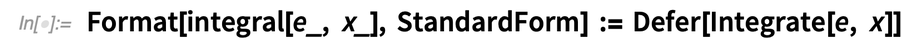✕

Using these rules, we can now integrate polynomials, for example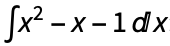: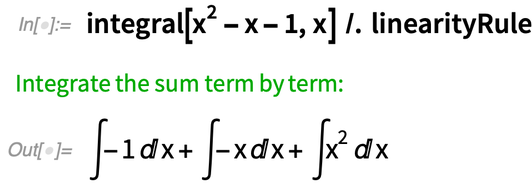✕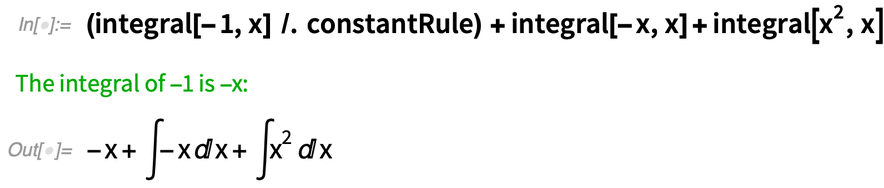✕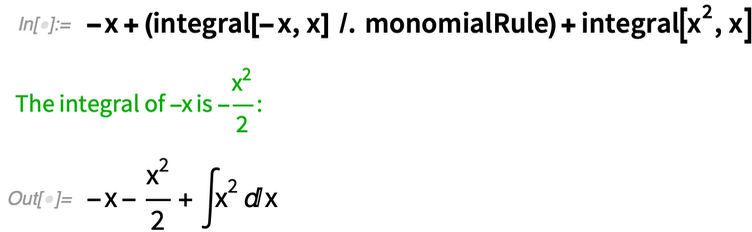✕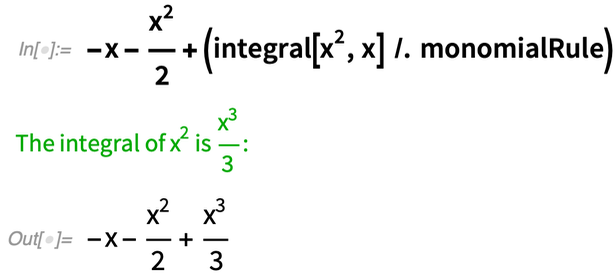✕

After spending far too many late nights entering integration techniques as pattern-matching rules into Mathematica, I had the code at a reasonable state and I sent it to Wolfram Research for possible inclusion in Mathematica. Soon after, I was offered a job and ended up working for Wolfram for several years, predominantly on Wolfram|Alpha. My step-by-step integrator is still computing many integrals, some of which I have most likely forgotten how to do myself.

As an aside, the idea of using rule-based programming to compute indefinite integrals dates back to 1961, with the Symbolic Automatic Integrator (SAINT) by James Slagle at MIT. SAINT could solve “symbolic integration problems approximately at the level of a good college freshman and, in fact, uses many of the same methods (including heuristics) used by a freshman” . The step-by-step integrator I wrote used around 350 rules and could integrate more than 99% of integrals in calculus textbooks. Since then, Albert Rich used Mathematica to create the Rule-based Integrator (Rubi), which uses over 6,700 rules.

Fast forward to 2020 and I hadn’t looked at integrals for a decade. I decided to see what advances had been made in the last 10 or so years. I was particularly interested in a Gröbner basis–based algorithm developed by Manuel Kauers and its extensions by Brian Miller that could seemingly outperform the algebraic case of the Risch algorithm in the AXIOM computer algebra system on many integrals [7, 8]. For example:✕

It’s trivial to check the result: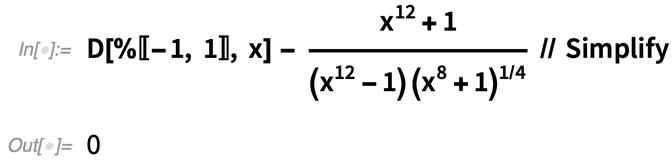✕

Once again, I quickly became hooked on integrals, or more specifically, algorithmic solutions to indefinite integrals.

When I last looked at symbolic integration, I was interested in the transcendental case of the Risch algorithm, of which Mathematica has a near-complete implementation. For example, the following is a simple integral for the transcendental case of the Risch algorithm: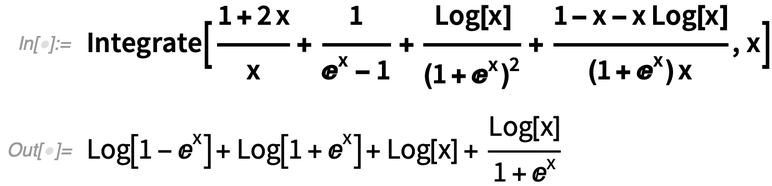✕

I became more interested with algebraic integrals, which cannot be integrated with the transcendental Risch algorithm. The algebraic case of the Risch algorithm is considerably more complex than the transcendental case and has not been completely implemented in any computer algebra system.

I initially considered an algebraic integral that appears in many calculus textbooks: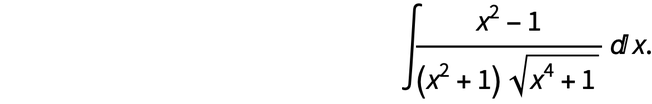✕

If we’re happy to play it fast and loose with branch cuts, then we can write this integral as: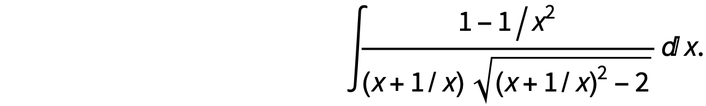✕

For this integral, we can substitute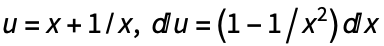and we get: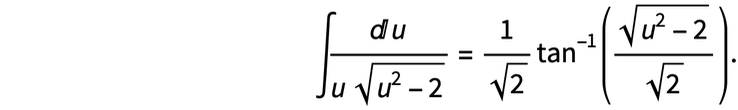✕

Substituting back, we get: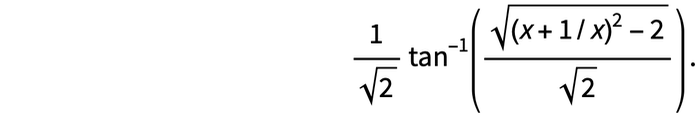✕

This answer has branch cut issues; however, we can fix this by writing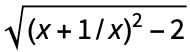as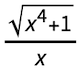. Then we have the correct antiderivative: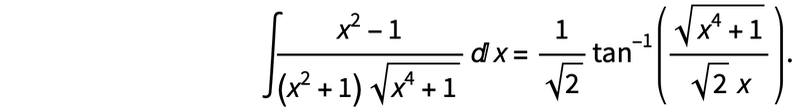✕

I wondered if this method of using a Laurent polynomial substitution to simplify an algebraic integral was just a trick that worked for this integral or a hint to a more general method. It turns out this trick works for many integrals; for example, the integral we tried previously on Kauers’s algorithm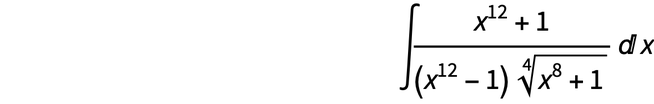✕

can be reduced to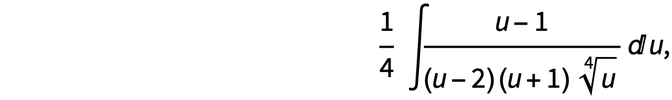✕

with the substitution u = x4 + x–4. Once corrected for branch cut issues, the solution is given by: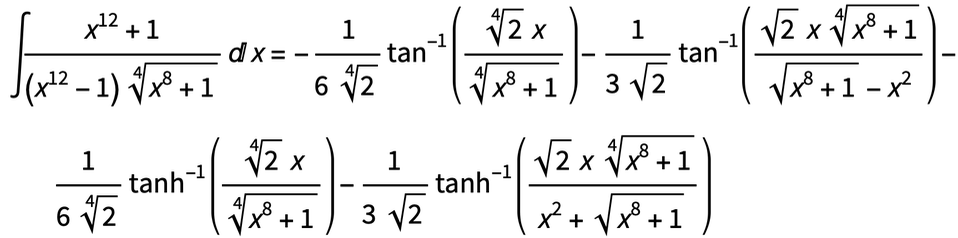✕

A general method would seek a substitution of the form u =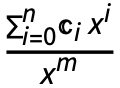such that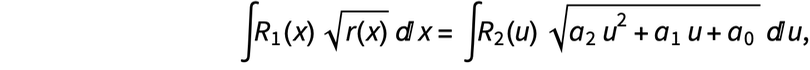✕

where R1(u), R2(u) are rational functions of u and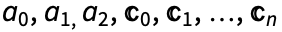are undetermined coefficients.

We start by using SolveAlways to compute the undetermined coefficients in the u substitution: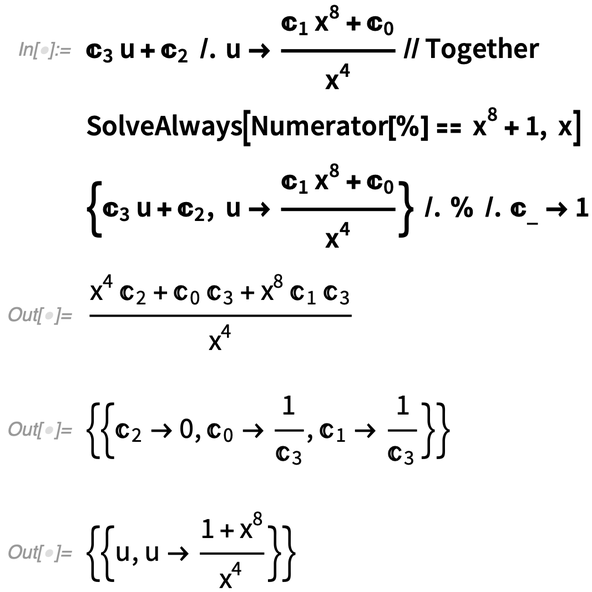✕

So we have a candidate substitution that fits the radicand part of the integrand. Does this substitution fit the rest of the integrand? We can compute this as follows: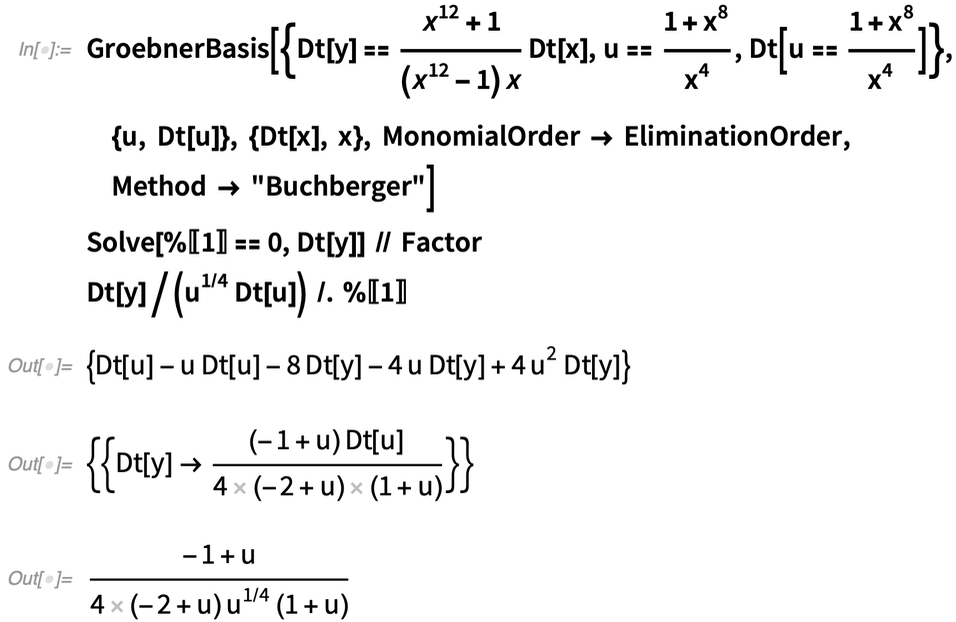✕

We have made the same simplification to the integral that we made by hand previously, namely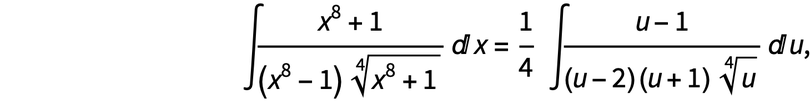✕

where u =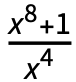.

This method is implemented with IntegrateAlgebraic at the Wolfram Function Repository. (In 2020, I further investigated the computation of pseudo-elliptic integrals in terms of elementary functions .) Given the simplicity of this method, it can integrate a wide range of integrals.

Here are some examples: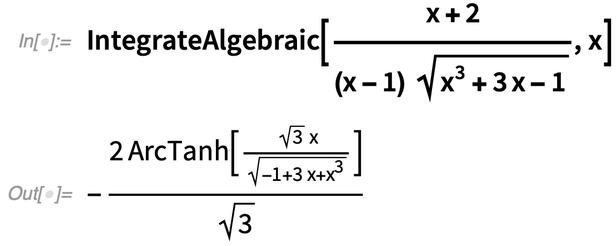✕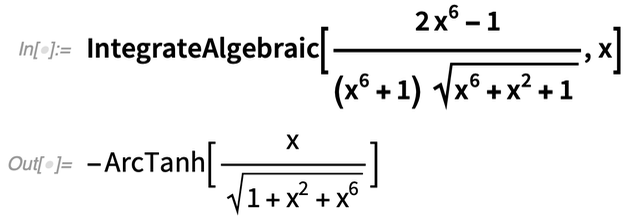✕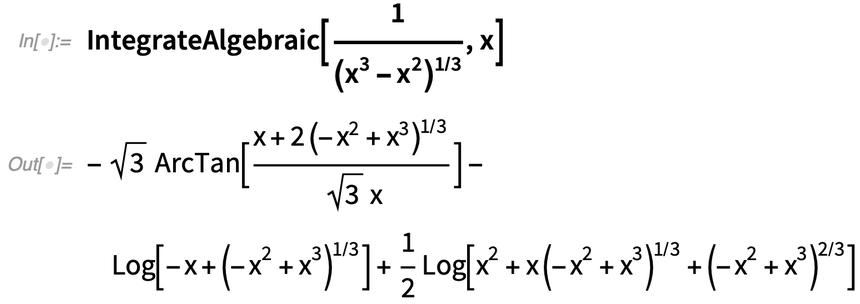✕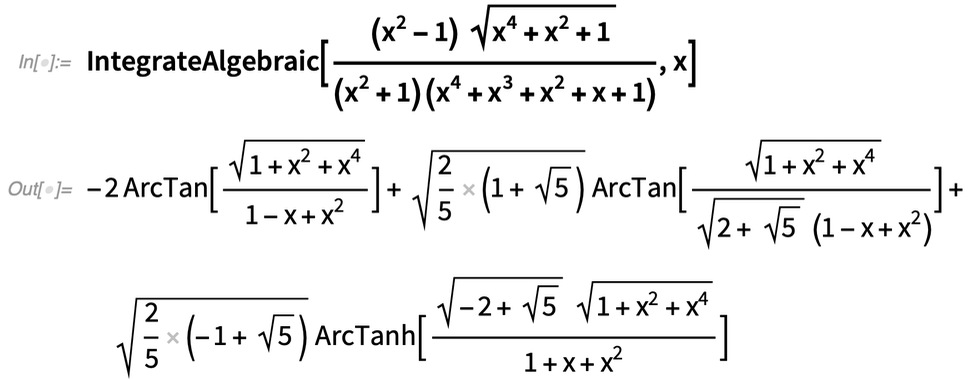✕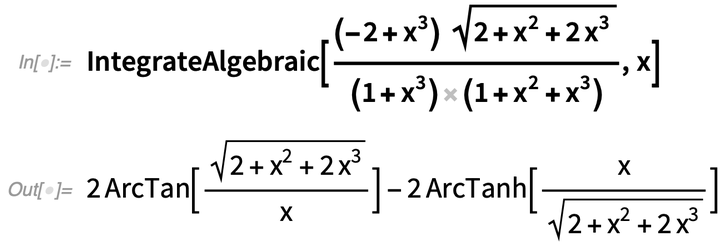✕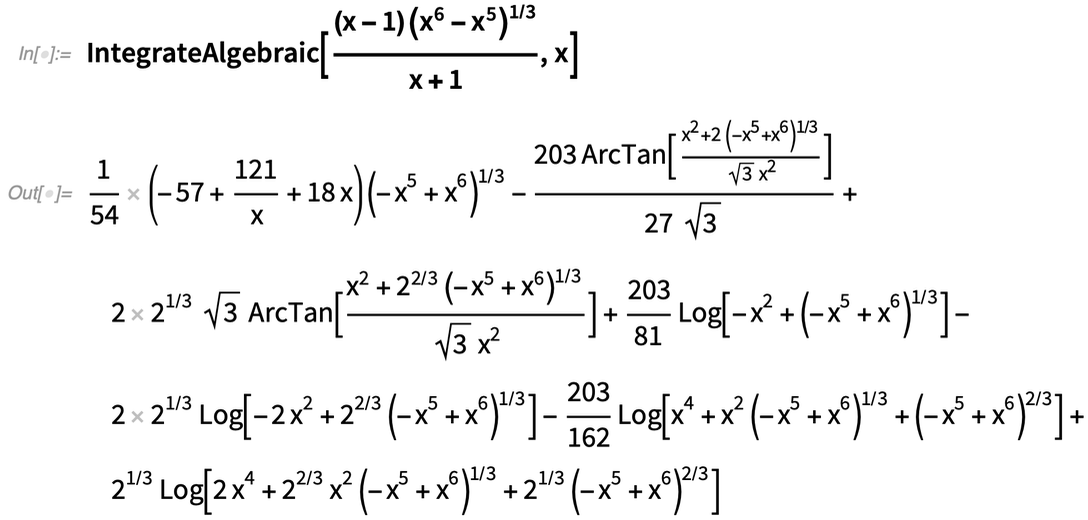✕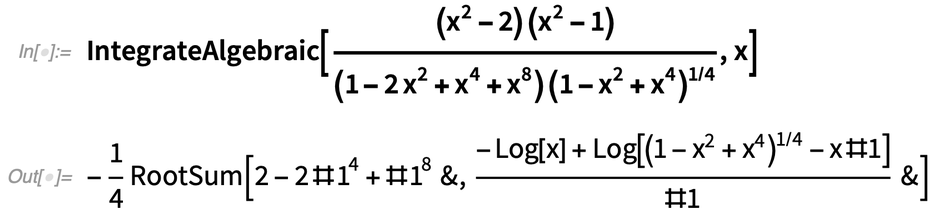✕

Unlike the algebraic case of the Risch algorithm, this technique can quickly solve many integrals involving parameters: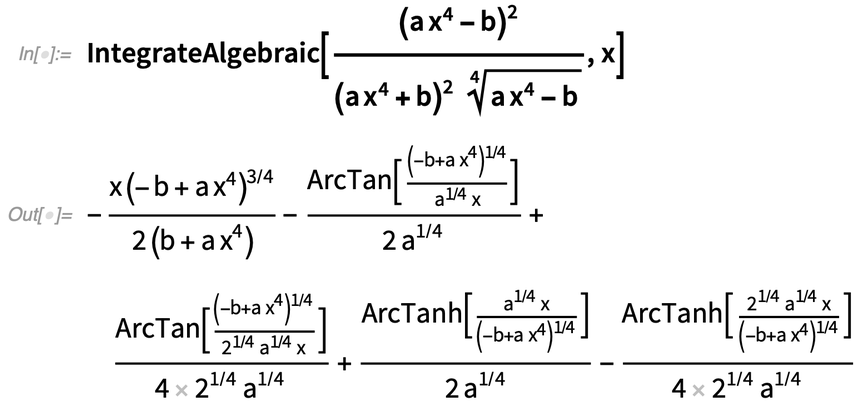✕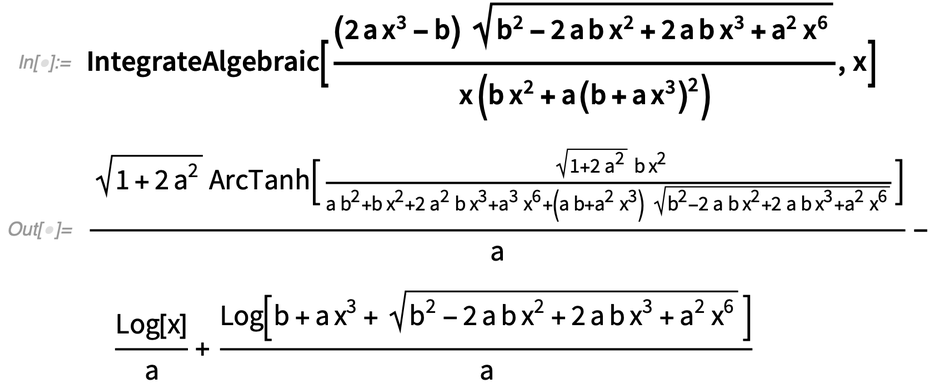✕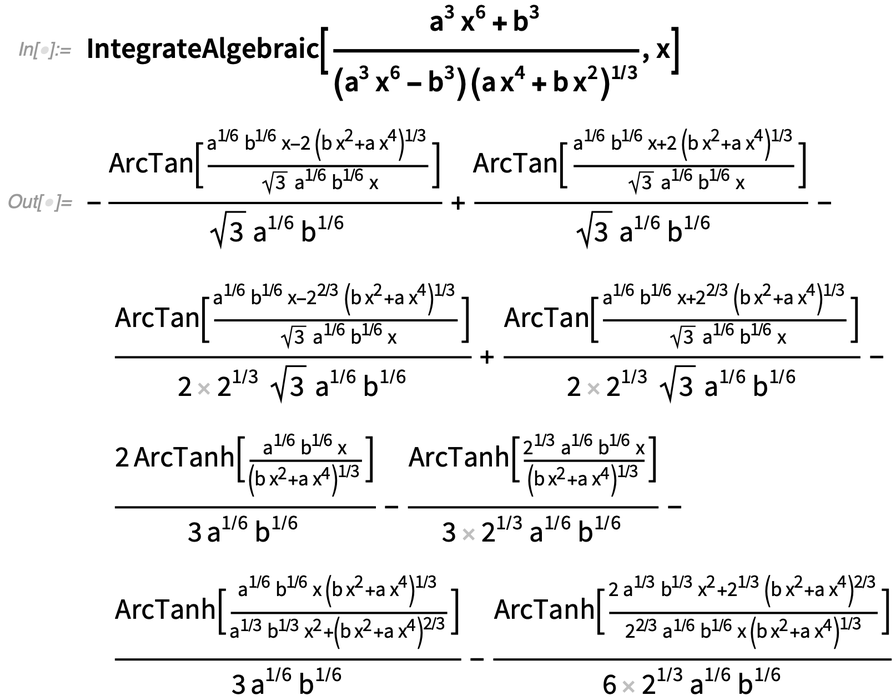✕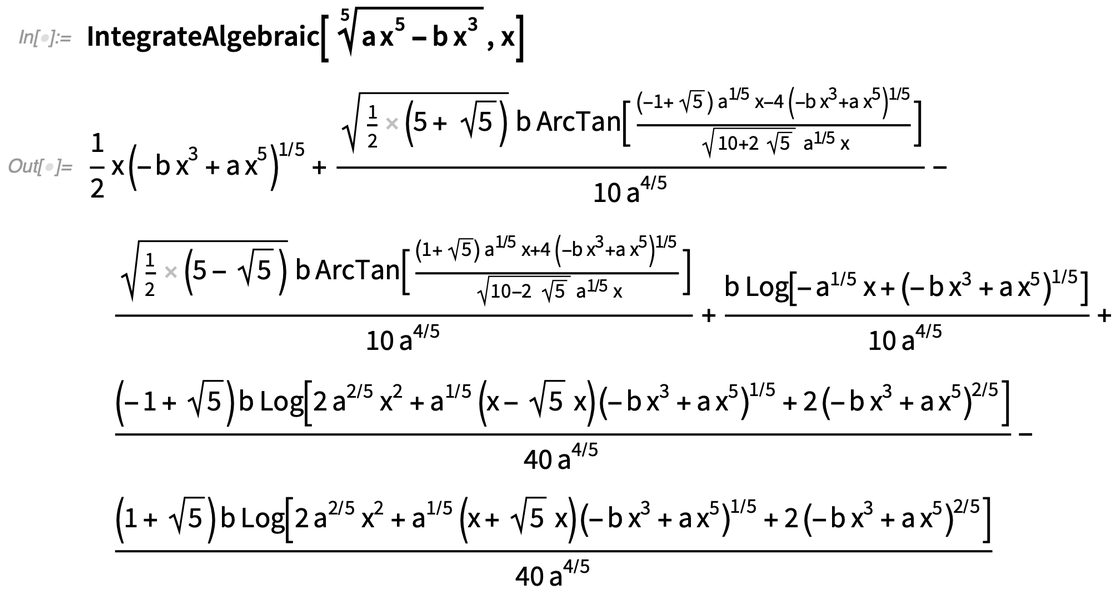✕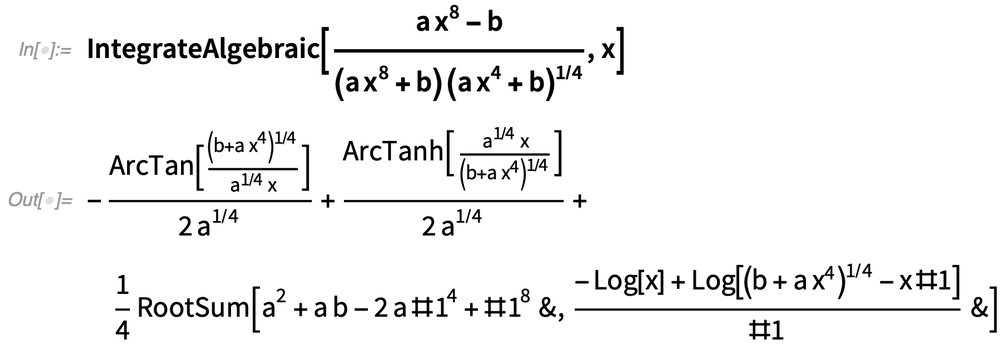✕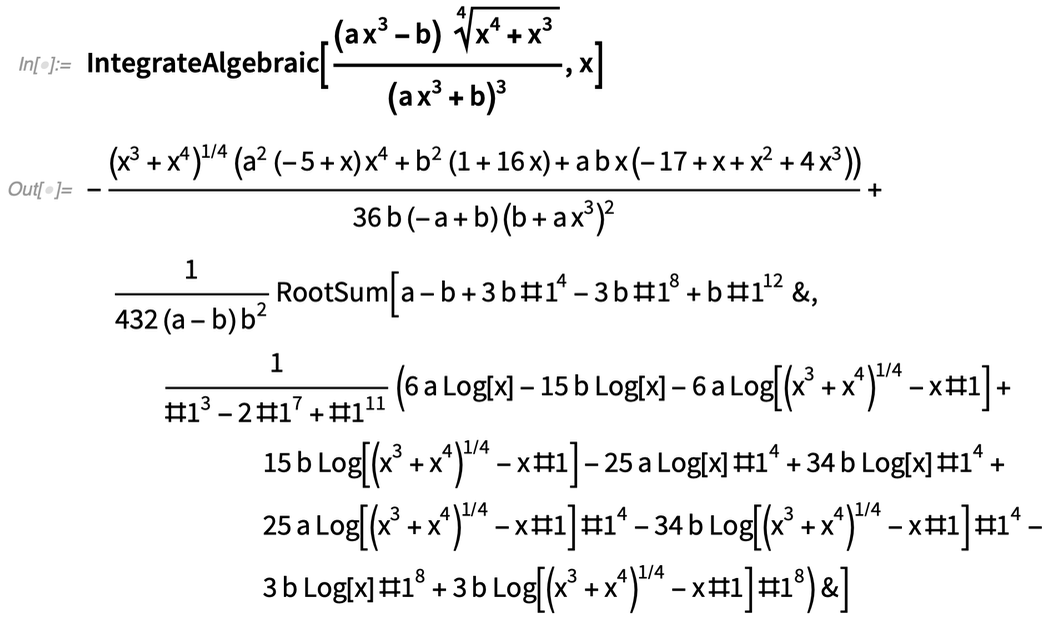✕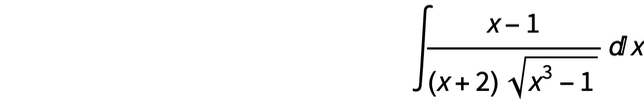✕

Unlike the previous examples, this integral is not solvable with a Laurent polynomial substitution.

In 1882, Günther developed a method for computing some otherwise difficult algebraic integrals . Given the integral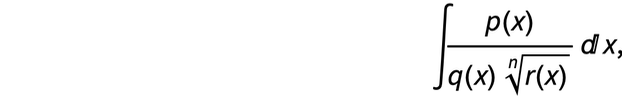✕

where p(x) and q(x) are polynomials in x, Günther made the substitution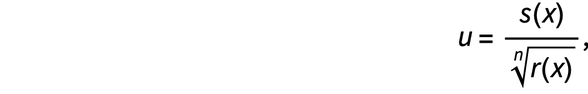✕

such that the integral becomes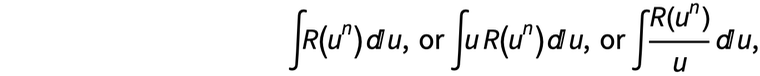✕

where s(x) = vQP+R–1 xQP+R–1 + vQP+R–2 xQP+R–2 + … + v1 x + v0, where P = degx(p(x)), Q = degx(q(x)), R = degx(r(x)) and v0, v1, …, vQP+R–1, c0, c1,c2, c3 are undetermined coefficients and R(un) is a rational function in un with undetermined coefficients.

We can use Günther’s method to solve this integral in Mathematica as follows. The substitution is of the form: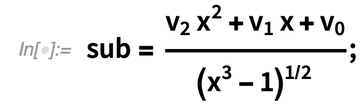✕

And we assume the integrand in u is of the form: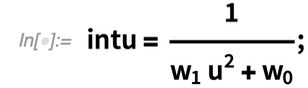✕

Then the integrand in x is given by: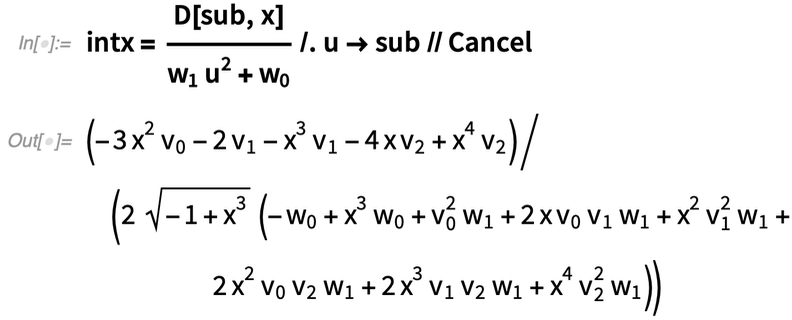✕

Now we need to solve for the undetermined coefficients in the substitution (v0, v1, v2) and in the rational integrand (w0, w1):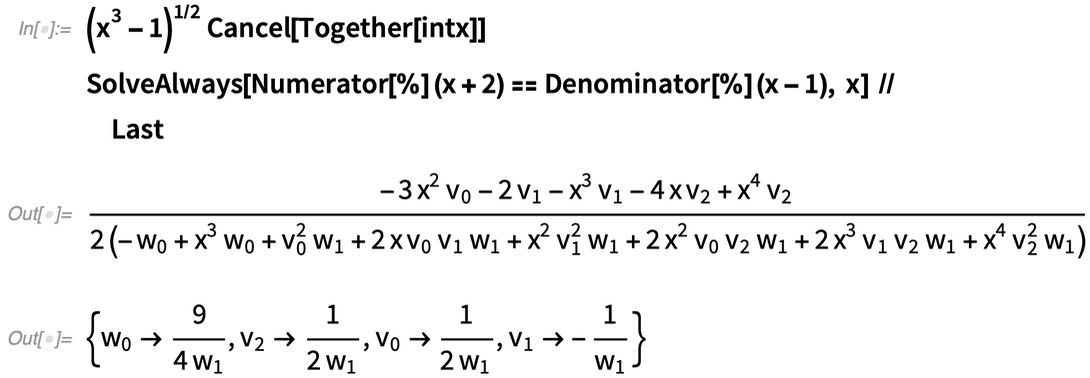✕

We can substitute this solution into our integrand in u and substitution: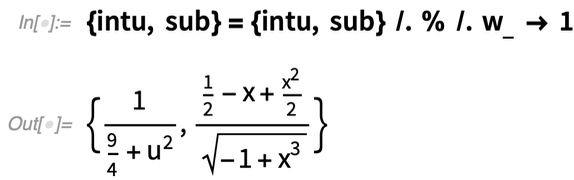✕

Now we can use Integrate to compute the resulting integral: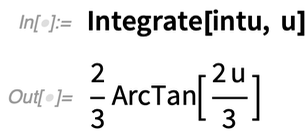✕

Then substitute back to solve our original integral: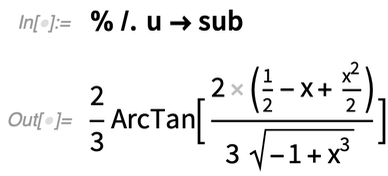✕

A quick check that our solution is correct: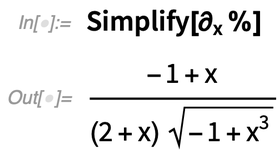✕

A generalized version of Günther’s method is implemented in IntegrateAlgebraic. This method can solve many otherwise difficult integrals. Here are some examples: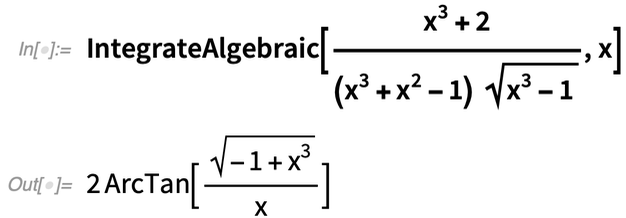✕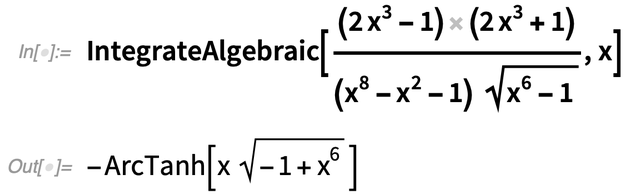✕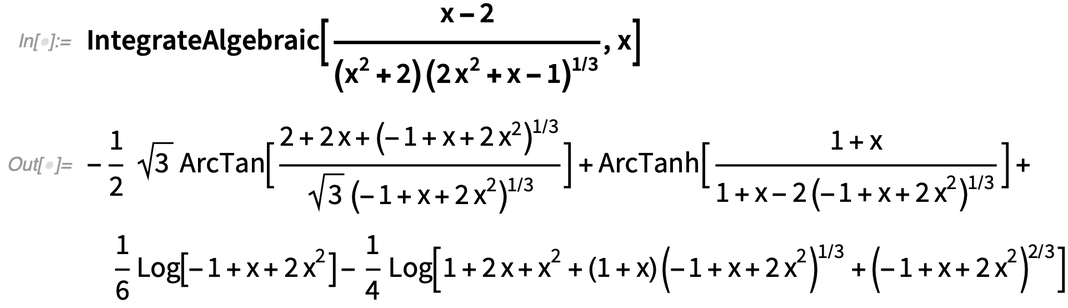✕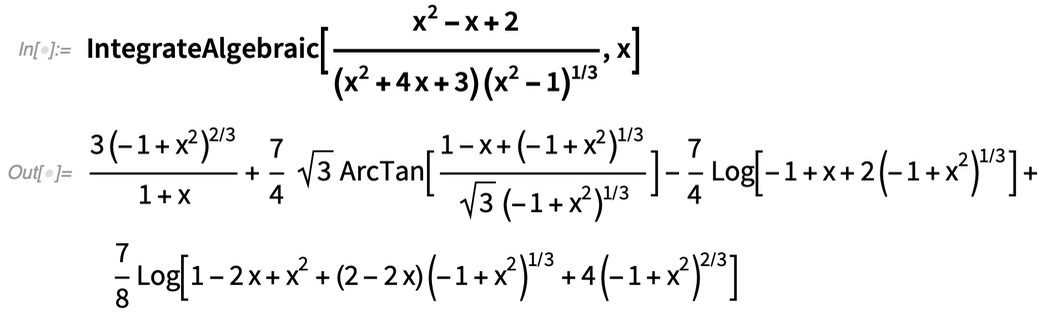✕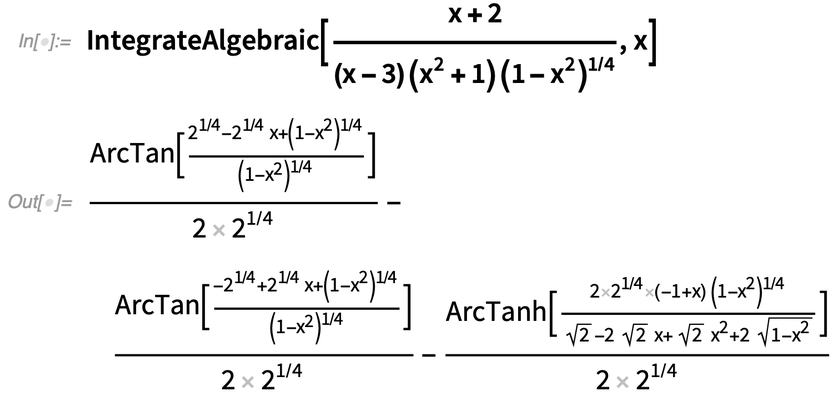✕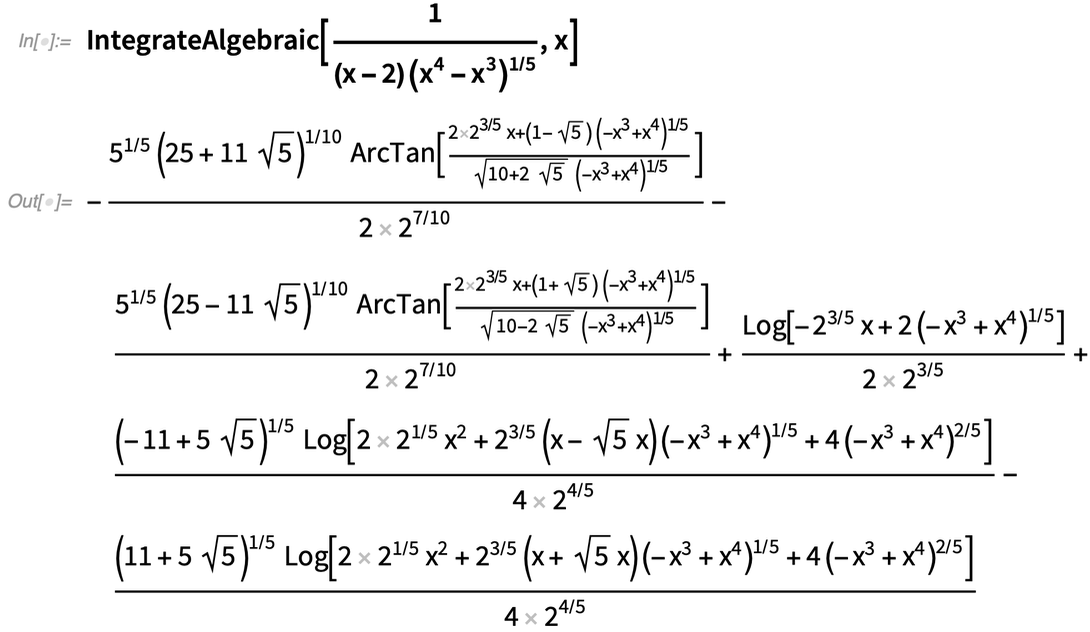✕

This method also handles integrals containing parameters: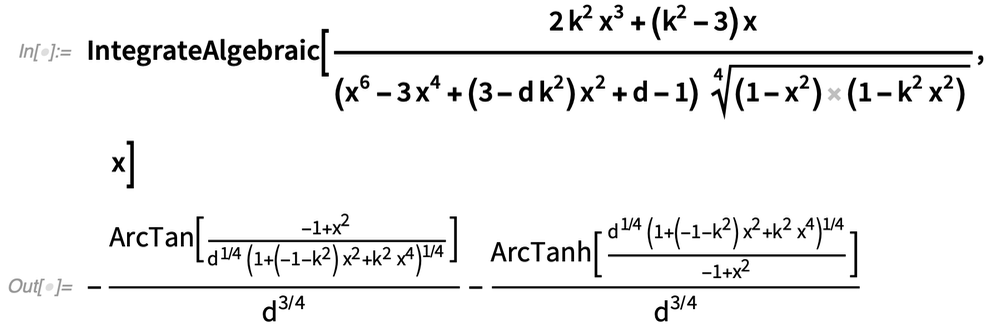✕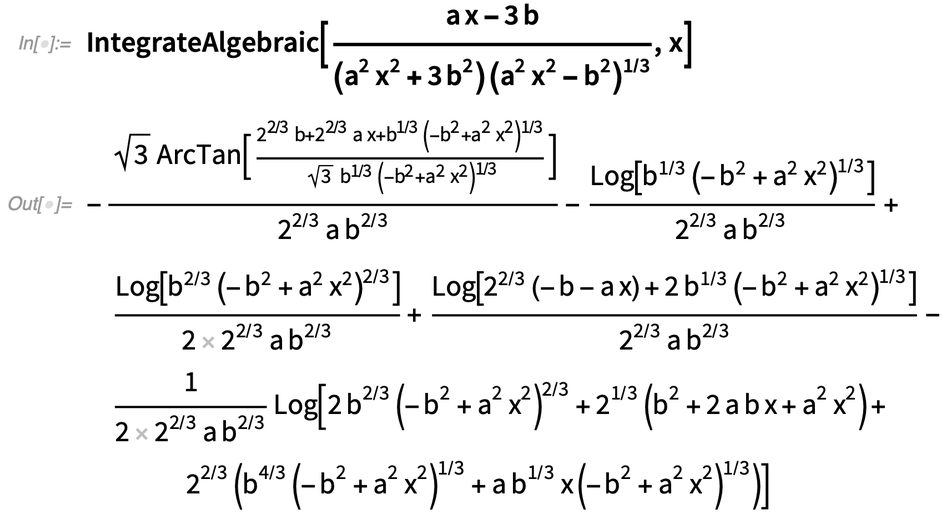✕

If we combine this method with integrating term by term after expanding the integrand into a sum of terms, we can handle more exotic algebraic integrals: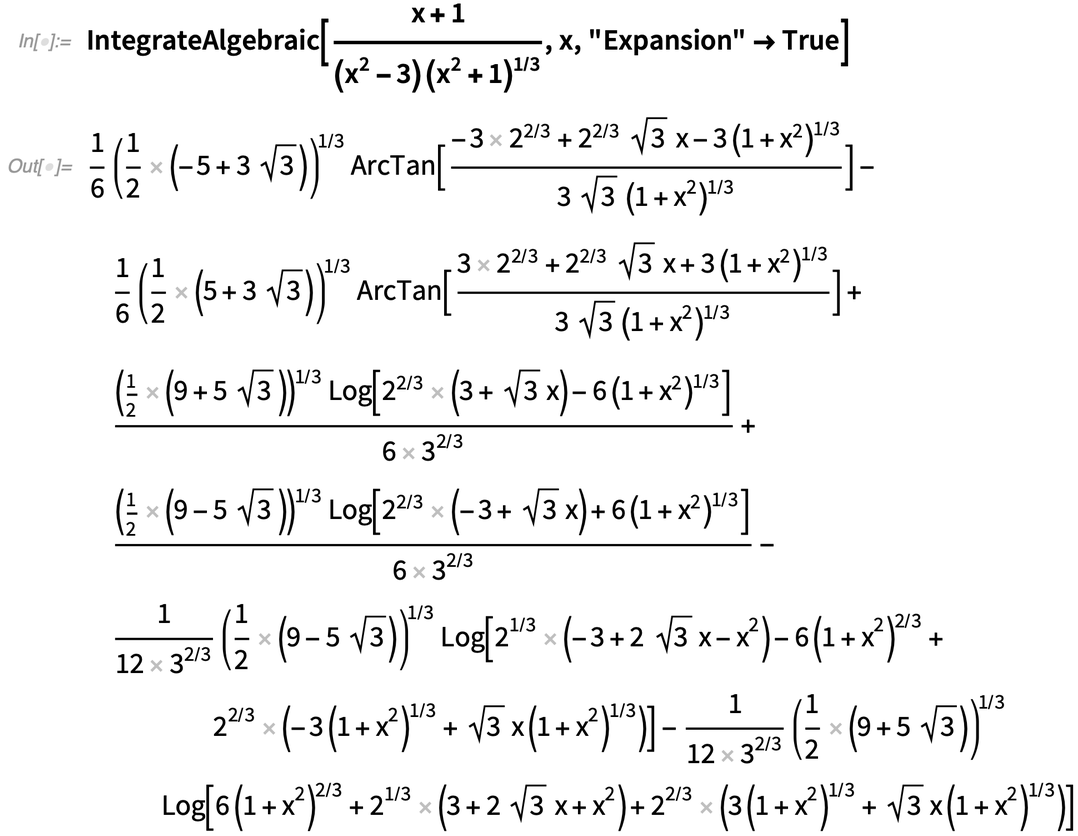✕

Combining the Laurent polynomial substitution method with the generalized Günther method and integrating term by term allows us to compute even more complex integrals: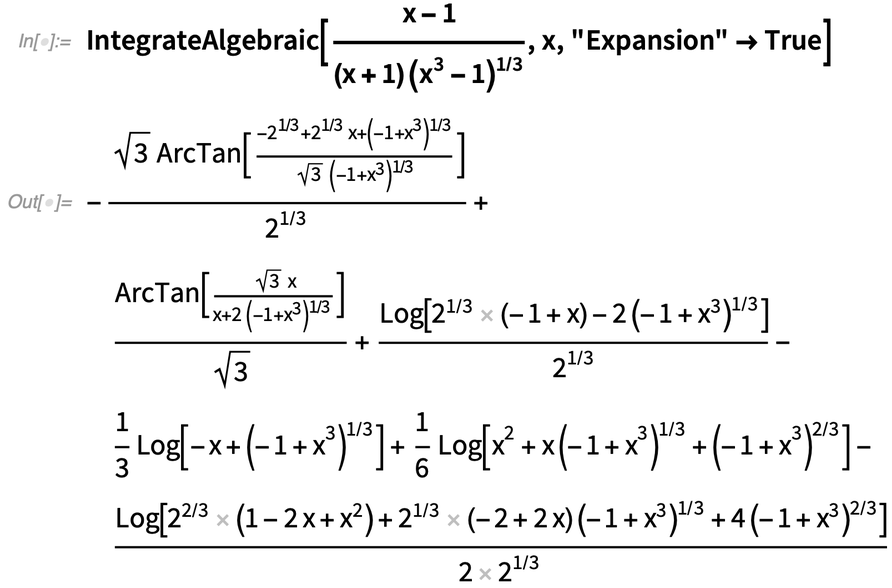✕

In this case, we wrote the integral as: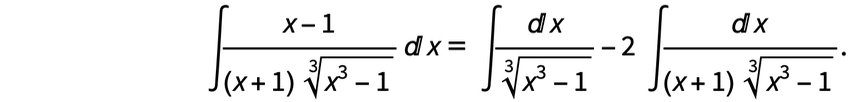✕

Then the integral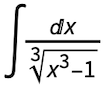was reduced to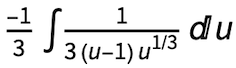with the substitution u = 1 – x3, while the integral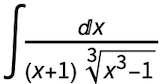was reduced to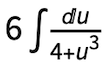with the substitution s =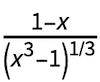.

Integrating expressions containing nested radicals has always been a tricky business. A well-known example is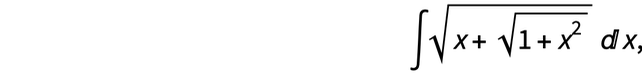✕

which can be computed using the substitution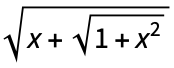. We can make this substitution with GroebnerBasis as follows: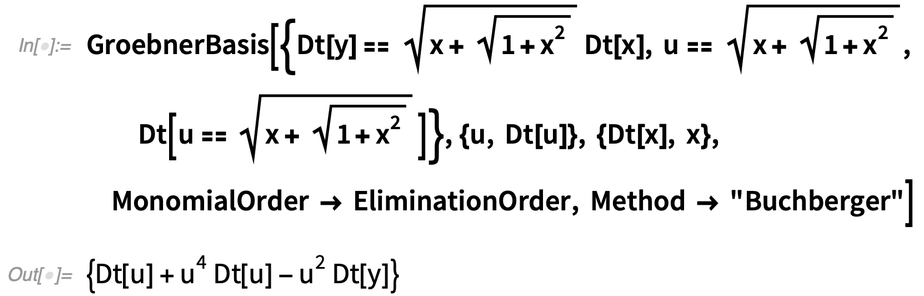✕

We need to express this relationship in terms of Dt[y]: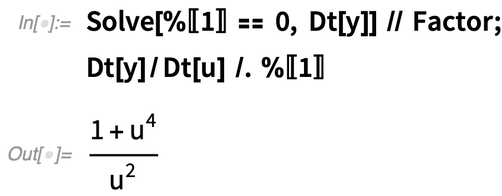✕

We can now integrate the rational function of u and substitute back for x: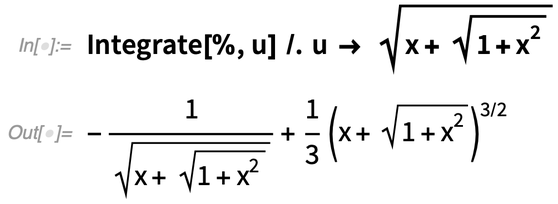✕

This method can solve much more difficult integrals involving nested radicals. For example: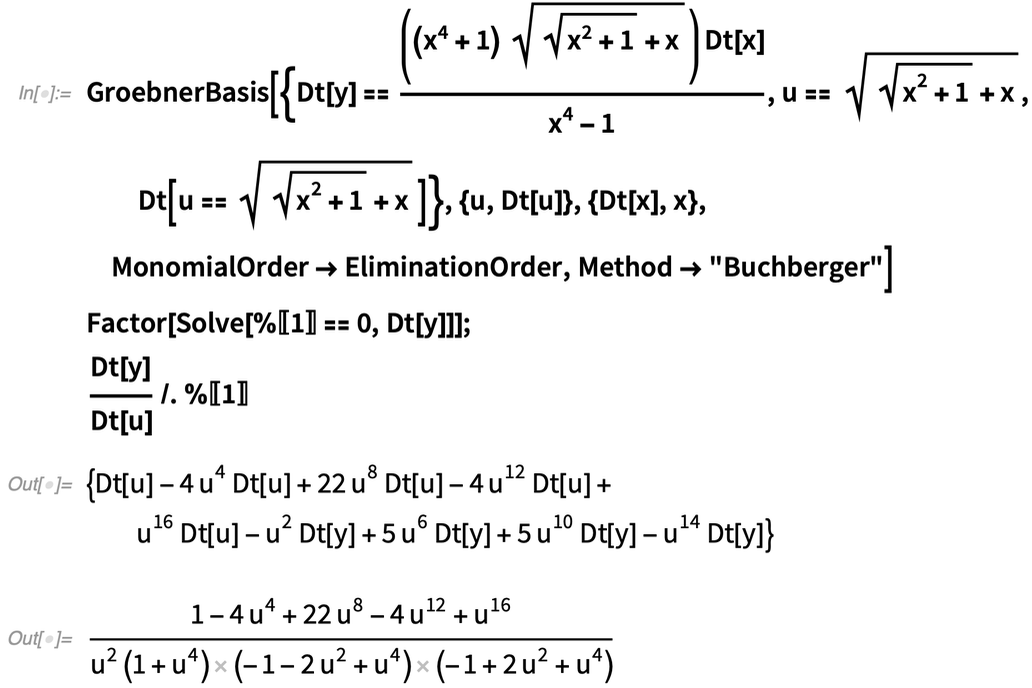✕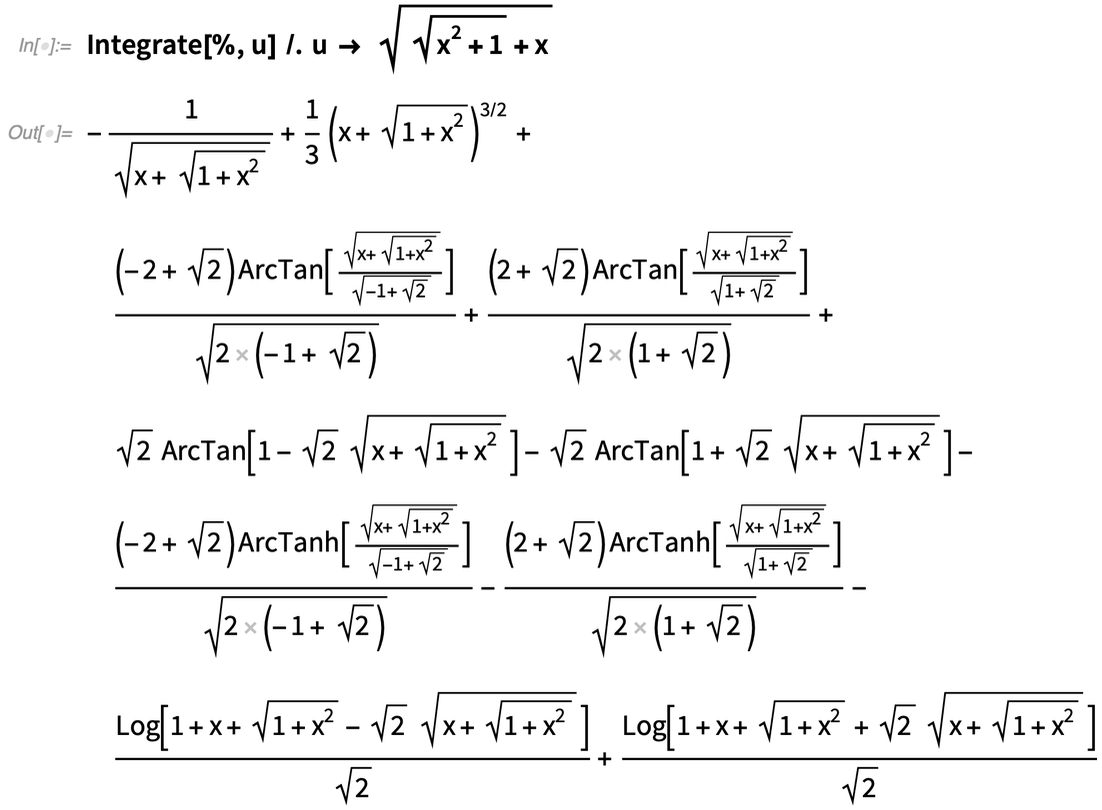✕

A generalization of this approach is used within IntegrateAlgebraic. Here are some challenging examples: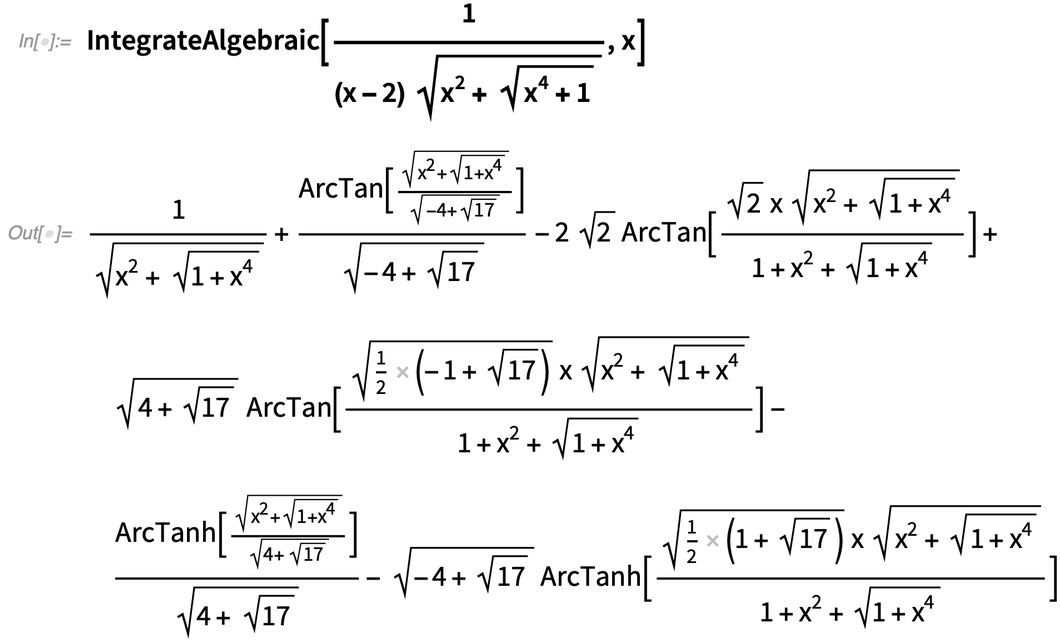✕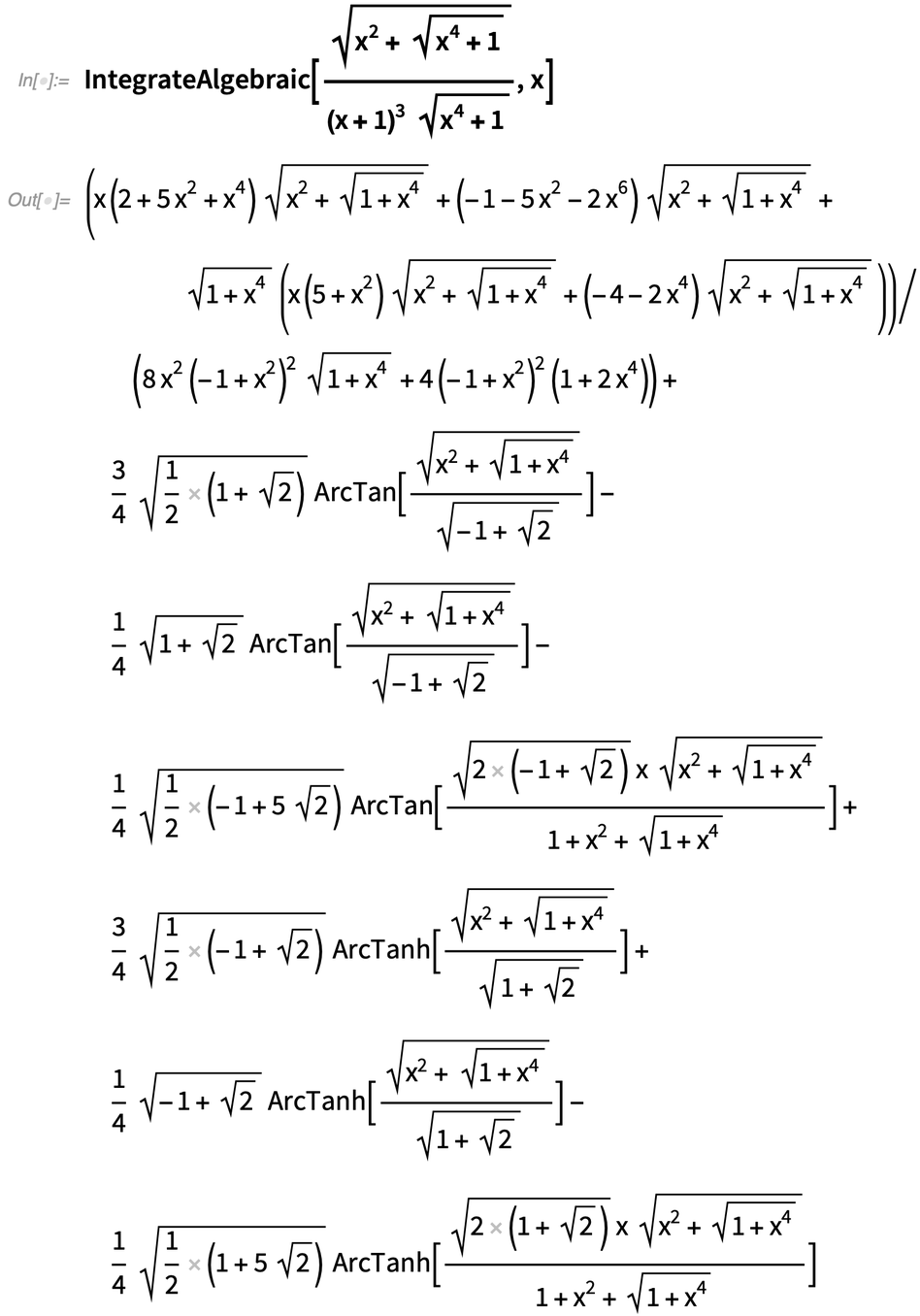✕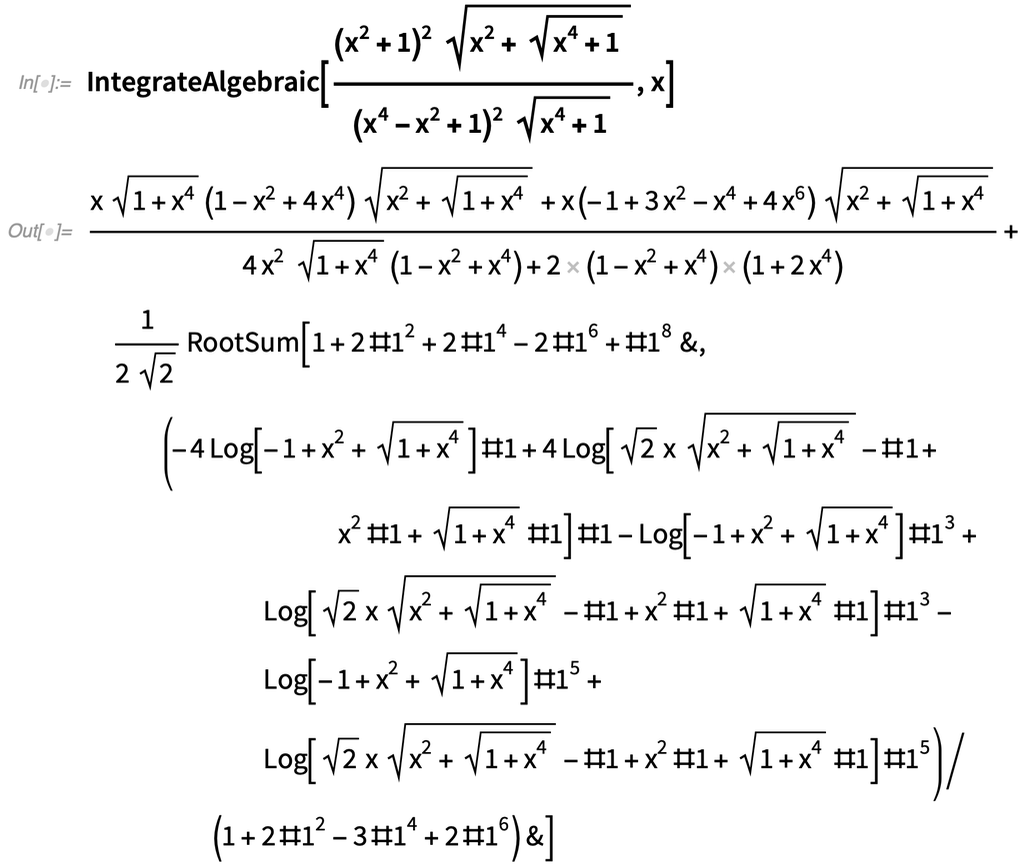✕

Like the other methods in IntegrateAlgebraic, we readily handle integrals involving parameters: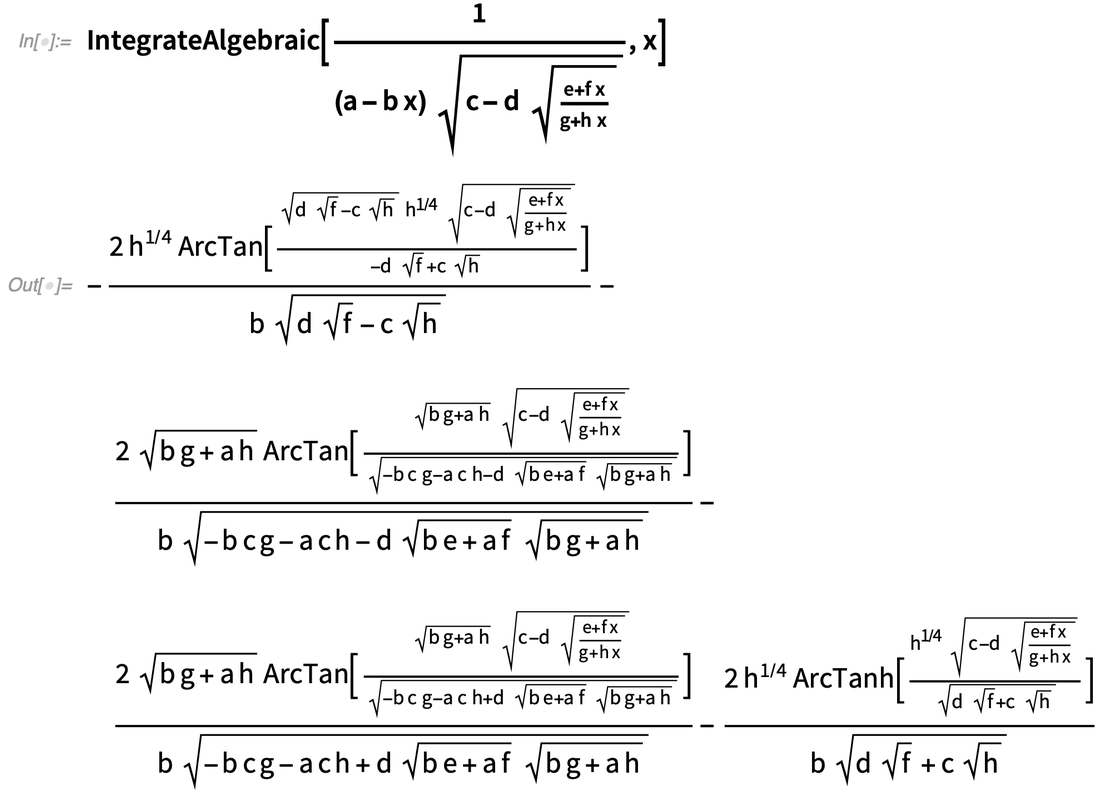✕

All of the integrals in this post contain polynomial radicands; however, these methods generalize to rational radicands. For example: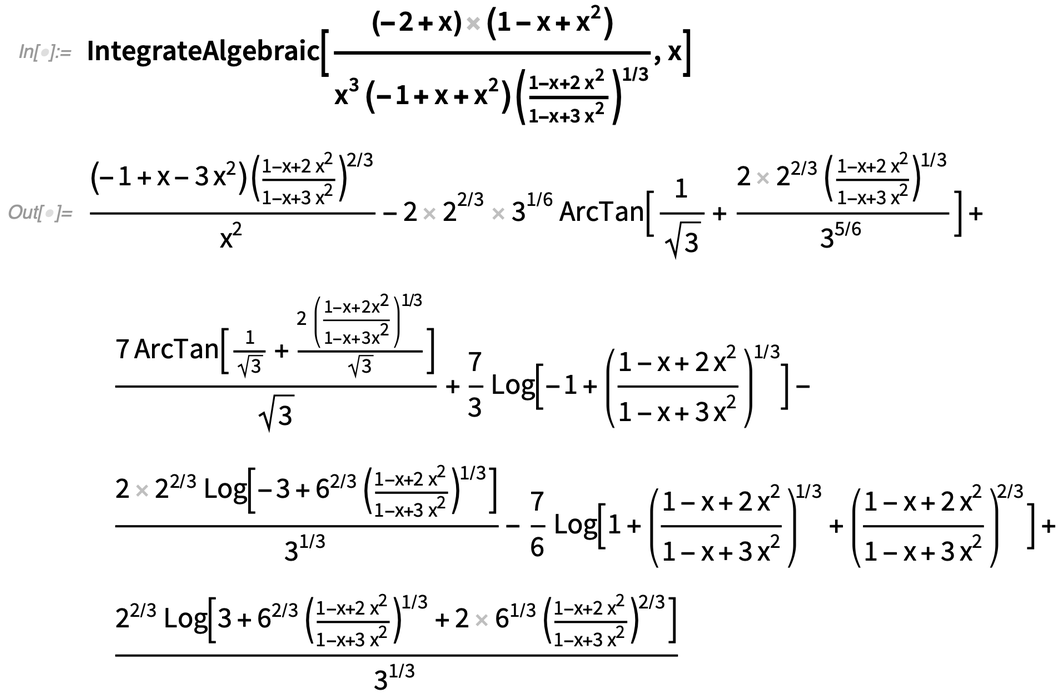✕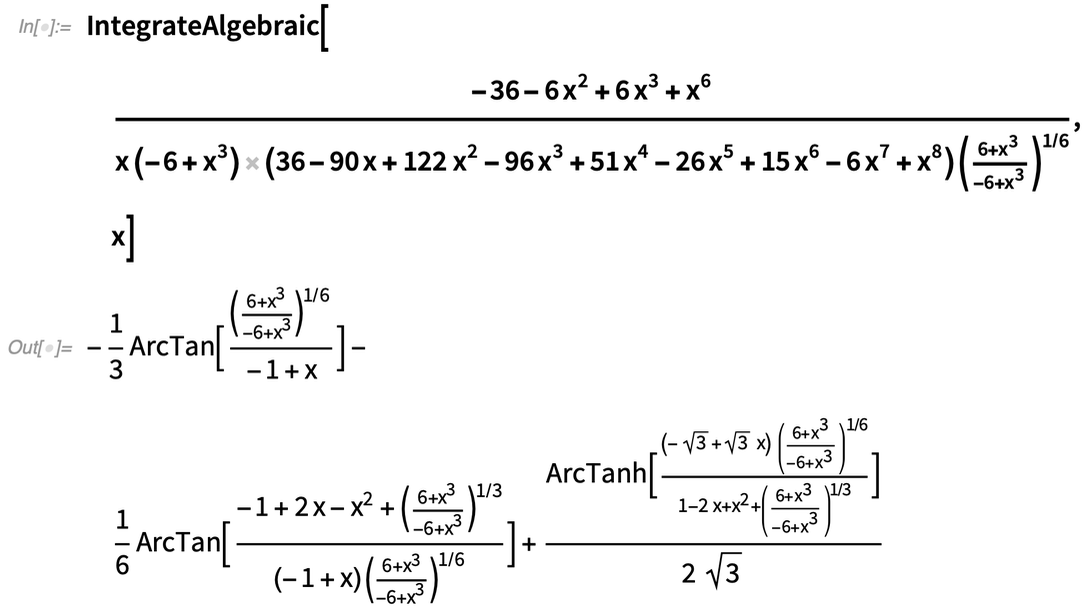✕

There are still many algebraic integrals that these methods will not compute. For example, the following integral possesses an elementary (albeit enormous) solution: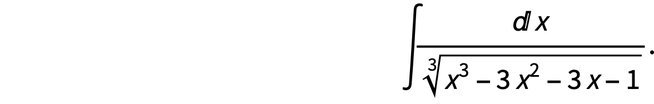✕

However, compared to the algebraic case of the Risch–Trager–Bronstein algorithm, which is not completely implemented in any computer algebra system, these methods are fast, simple and complement the existing integration capabilities of Mathematica’s Integrate function. We are currently considering including IntegrateAlgebraic within Integrate in an upcoming release.

 R. Risch, “The Problem of Integration in Finite Terms,” American Mathematical Society, 139(1), 1969 pp. 167–189.

 R. Risch, “The Solution of the Problem of Integration in Finite Terms,” Bulletin of the American Mathematical Society, 76(3), 1970 pp. 605–608.

 J. Davenport, “Integration of Algebraic Functions,” in EUROSAM ’79: Proceedings of the International Symposium on on Symbolic and Algebraic Computation, 1979 pp. 415–425.

 M. Bronstein, “Integration of Elementary Functions,” Journal of Symbolic Computation, 9(2), 1990 pp. 117–173.

 B. Trager, “Integration of Algebraic Functions,” dissertation, Massachusetts Institute of Technology, Dept. of Electrical Engineering and Computer Science, 1984.

 J. Slagle, “A Heuristic Program That Solves Symbolic Integration Problems in Freshman Calculus: Symbolic Automatic Integrator (SAINT),” dissertation, Massachusetts Institute of Technology, Dept. of Mathematics, 1961.

 M. Kauers, “Integration of Algebraic Functions: A Simple Heuristic for Finding the Logarithmic Part,” ISSAC ’08, 2008 pp. 133–140.

 B. Miller, “On the Integration of Elementary Functions: Computing the Logarithmic Part,” dissertation, Texas Tech University, Dept. of Mathematics and Statistics, 2012.

 S. Blake, “A Simple Method for Computing Some Pseudo-elliptic Integrals in Terms of Elementary Functions,” 2020. arxiv.org/abs/2004.04910.

 S. Günther, “Sur l’évaluation de certaines intégrales pseudo-elliptiques,” Bulletin de la Société Mathématique de France, 10, 1882 pp. 88–97. www.numdam.org/article/BSMF_1882__ 10__ 88_ 1.pdf.

 Get full access to the latest Wolfram Language functionality with a Mathematica 12.3 or Wolfram|One trial.